# Pedro Tavares, Paulo Tabuada, ‡

 Fig. 5 - Earth's Geomagnetic Field - Simulation The results performance of the simulator was described in (Tavares, 1998). Control Algorithms Several researchers from various countries have already begun to explore and solve the control problems imposed by a LEO small satellite. (Ong, 1992) proposes some intuitive control laws to tackle this problem, but the actuation is very restricted and does not take advantage of the time-varying nature of this problem. (Steyn, 1994) approaches the control problem by using a Fuzzy Logic Controller that achieves better results than a Linear Quadratic Regulator (LQR), despite considering the constraint of actuating on a single coil at each actuation time. This approach suggests that non-linear and time-varying control methodologies should be further explored so that a better problem understanding and possible solutions may be found. (Wisniewski, 1996) compares two non-linear solutions: sliding mode control and energy based control, achieving better results than LQRs based on linear periodic theory. Using the ConSat simulator as a workbench, several attitude control algorithms were implemented and preliminary tests carried out, without the use of the attitude determination block in the loop. Two different control objectives were studied: attitude stabilisation and attitude stabilisation with spin control. In the first group, the present PoSAT-1 controller, a sliding mode controller, an energy-based controller and a predictive regulator were studied. In the second group the existing spin controller for PoSAT-1, an energy-based controller, a fuzzy logic controller and, finally, a predictive controller were studied. The above-mentioned algorithms, with the exception of the predictive controller, are described in detail in (Ong, 1992), (Steyn, 1994) and (Wisniewski, 1996). The predictive algorithm developed is a new algorithm for attitude stabilisation and spin control of small satellites using only electromagnetic actuation. This approach takes advantage of the time varying nature of the problem (the geomagnetic field changes through the orbit) by using the most appropriate control effort (according to an energy-based criterion) given the geomagnetic field and the satellite angular velocity at each actuation instant. Problem formulation The dynamics of a small satellite are well known and may be expressed in the Control CS as (Wertz, 1995):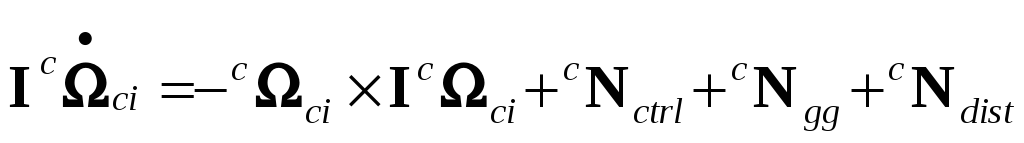(1) where I is the inertia tensor,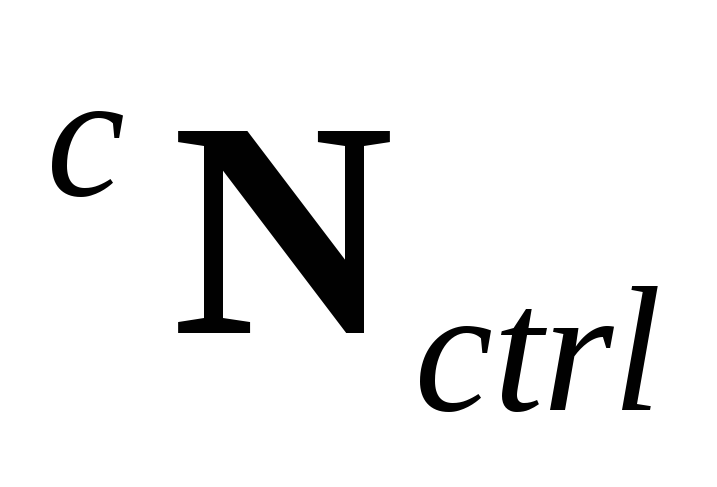the control torque,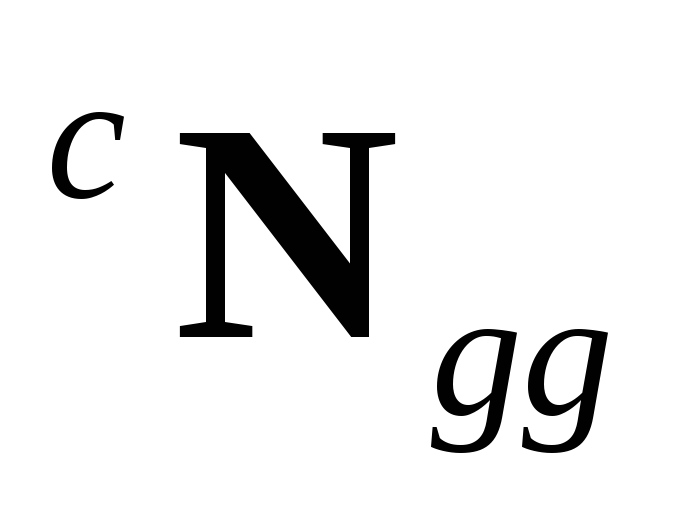the gravity gradient torque and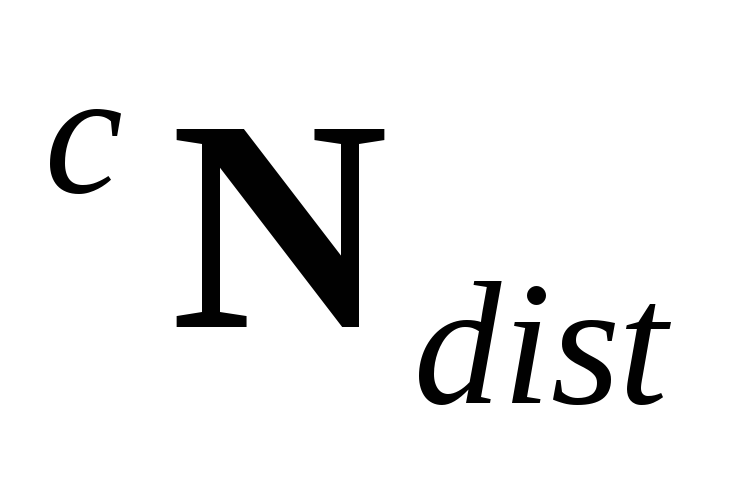a disturbance torque cause by aerodynamic drag and other effects.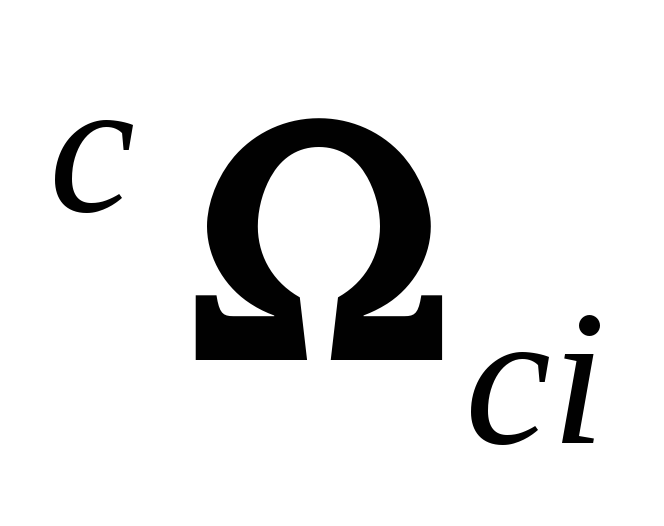is the angular velocity of the Control CS w.r.t. the Inertial CS written in the Control CS. Download 1.52 Mb.Share with your friends: